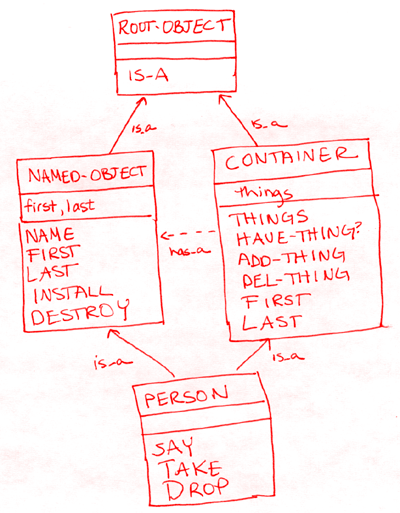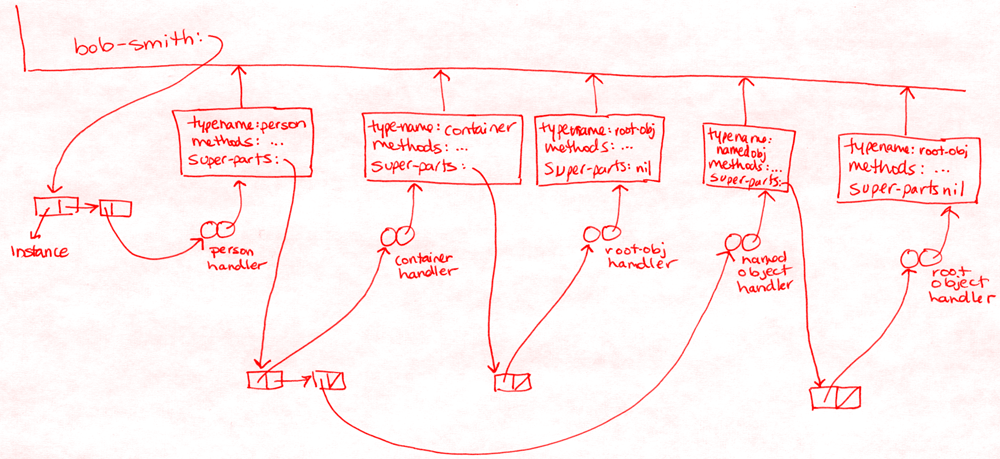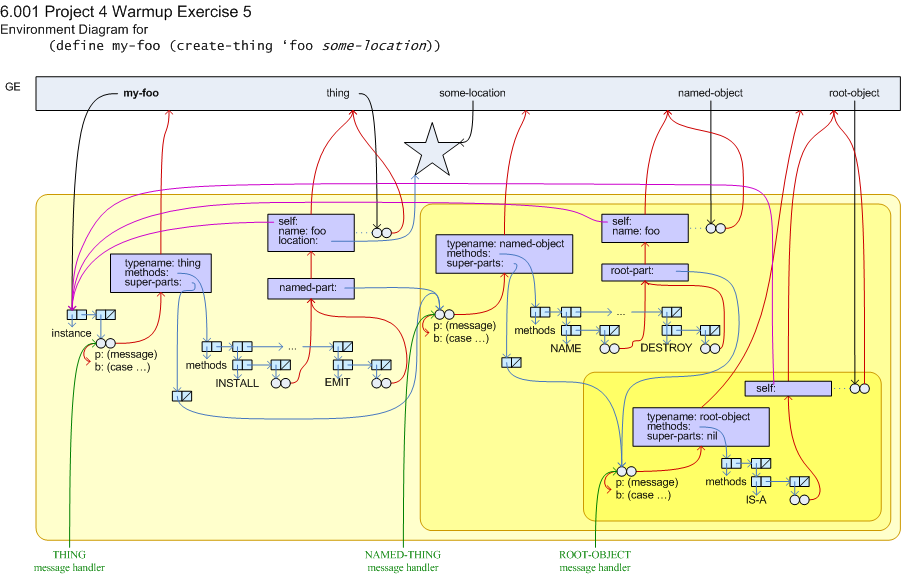```recitation 17: object-oriented programming

========================================================================

* class: the common structure of a type of object
* instance: a particular object of a given class
* inheritance: a subclass can extend the functionality
of one or more superclasses
* "is-a": given a generic object, you can investigate its type,
an object "is-a" anything in its inheritance hierarchy.
* "has-a": an object may store pointers to other objects
as part of its state.

* shadowing of methods, objects as "frames" -- lookup method/variable by
following up a chain and finding first handler for that message
* if there's a method that does what you want, use it!
(not using it is an abstraction violation)
* template for class definitions
* asking self to do something vs. asking a part to do something
* create-book vs. book: why do some objects have a create-THING & a THING
procedure and other objects just have a THING procedure?

========================================================================
exercises (refer to the code below)

* draw the inheritance hierarchy for the code below.* draw the environment diagram for:
(define bob-smith (create-person 'bob 'smith))The above diagram shows just the multiple inheritance relationships
between the classes.  Note how there are two root object handlers!
The diagram below for Project warmup exercise #5, by TA Gerald Dally,
is a more complete illustration of the workings of our object system.* finish the code for person's TAKE method, use display to print stuff.
people can't take things they already have, and they can't take other
people.
(define bob-smith (create-person 'bob 'smith))
(define jane-doe (create-person 'jane 'doe))
(define ice-cream (create-named-object 'chocolate 'swirl))
see code below

========================================================================
MODIFIED CODE FROM LECTURE / PROJECT 4

;; root-object
(define (root-object self)
(make-handler
'root
(make-methods
'IS-A
(lambda (type)

;; named-object
(define (create-named-object first last)
(create-instance named-object first last))
(define (named-object self first last)
(let ((root-part (root-object self)))
(make-handler
'named-object
(make-methods
'NAME    (lambda () first)
'FIRST   (lambda () first)
'LAST    (lambda () last)
'INSTALL (lambda () 'installed)
'DESTROY (lambda () 'destroyed))
root-part)))

;; container
(define (container self)
(let ((root-part (root-object self))
(things '()))
(make-handler
'container
(make-methods
'THINGS      (lambda () things)
'FIRST	   (lambda () (if (null? things) #f (car (reverse things))))
'LAST	   (lambda () (if (null? things) #f (car (reverse things))))
'HAVE-THING? (lambda (thing)
(not (null? (memq thing things))))
(if (not (ask self 'HAVE-THING? thing))
(set! things (cons thing things)))
'DONE)
'DEL-THING   (lambda (thing)
(set! things (delq thing things))
'DONE))
root-part)))

;; person
(define (create-person first last)
(create-instance person first last))
(define (person self first last)
(let ((named-object-part (named-object self first last))
(container-part    (container self)))
(make-handler
'person
(make-methods
'SAY (lambda (list-of-stuff) (for-each display list-of-stuff) (newline) 'said)
'TAKE (lambda (thing)
(ask self 'SAY (list "I try but cannot take " (ask thing 'NAME))))
(else
'DROP (lambda (thing)
(display "I drop ")
(newline)
container-part
named-object-part)))

========================================================================
CODE FROM LECTURE / PROJECT  --  NOT MODIFIED

;; Instance
(define (make-instance)
(list 'instance #f))
(define (instance? x)
(and (pair? x) (eq? (car x) 'instance)))
(define (set-instance-handler! instance handler)
(set-car! (cdr instance) handler))
(define (create-instance maker . args)
(let* ((instance (make-instance))
(handler (apply maker instance args)))
(set-instance-handler! instance handler)
(if (method? (get-method 'INSTALL instance))
instance))

;; Handler maker helper
(define (make-handler typename methods . super-parts)
(cond ((not (symbol? typename))    ;check for possible programmer errors
((not (method-list? methods))
((and super-parts (not (filter handler? super-parts)))
(else
(named-lambda (handler message)
(case message
((TYPE)
(lambda () (type-extend typename super-parts)))
((METHODS)
(lambda ()
(append (method-names methods)
(append-map (lambda (x) (ask x 'METHODS))
super-parts))))
(else
(let ((entry (method-lookup message methods)))
(if entry
(find-method-from-handler-list message super-parts)))))))))

(define (make-methods . args)
(define (helper lst result)
(cond ((null? lst) result)
; error catching
((null? (cdr lst))
(error "unmatched method (name,proc) pair"))
((not (symbol? (car lst)))
(if (procedure? (car lst))
(pp (car lst)))
(error "invalid method name" (car lst)))
(error "invalid method procedure" (cadr lst)))
(else
(helper (cddr lst) (cons (list (car lst) (cadr lst)) result)))))
(cons 'methods (reverse (helper args '()))))
(define (method-list? methods)
(and (pair? methods) (eq? (car methods) 'methods)))
(define (empty-method-list? methods)
(null? (cdr methods)))
(define (method-lookup message methods)
(assq message (cdr methods)))
(define (method-names methods)
(map car (cdr methods)))

;; Method Interface
(define (ask object message . args)
(let ((method (get-method message object)))
(cond ((method? method)
(apply method args))
(else
(error "No method for" message 'in
object 'NAME))))))

(define (get-method message . objects)
(find-method-from-handler-list message (map ->handler objects)))
(define (find-method-from-handler-list message objects)
(if (null? objects)
(no-method)
(let ((method ((car objects) message)))
(if (not (eq? method (no-method)))
method
(find-method-from-handler-list message (cdr objects))))))

; used in make-handler to build the TYPE method for each handler
(define (type-extend type parents)
(cons type
(remove-duplicates
(append-map (lambda (parent) (ask parent 'TYPE))
parents))))

========================================================================
miscellaneous new stuff:

* write a procedure foo that takes in a symbol ('one 'two 'three ... )
and returns the integer it maps to (1 2 3).  use case.

(foo 'one) ==> 1
(foo 'two) ==> 2
(foo 'three) ==> 3

(define (foo sym)
(case sym
((one) 1)
((two) 2)
((three) 3)
(else (error "unknown sym" sym))))

* write a procedure truncate-lists! that takes in a list of lists and
modifies each sublist so it only has the first element.  use for-each.
for-each is like map except it throws away the result.  so op must be
executed for it's side effect...

(define thing (list (list 1 2 3 4) (list 5 6) (list 7)))
thing ==>  ((1 2 3 4) (5 6) (7))
(truncate-lists! thing)
thing ==>  ((1) (5) (7))

(define (truncate-lists! lsts)
(for-each (lambda (x) (set-cdr! x nil)) lsts))

* named-let: used in the project code.  it's in the scheme manual, but
you don't need to know it.

(let loop ((count 5))
(if (> count 0)
(begin (display count)
(loop (- count 1)))
'done))

* named-lambda: also used in the project code.  it's not defined in
dr. scheme so you may need to work in the lab for this project.  sorry.

(named-lambda (handler message)
(case message
((TYPE) ... )))